# 《基于事件流的高效模式匹配》论文中文版

Flink的CEP实现重度参考了论文《Efficient Pattern Matching over Event Streams》，此文是该论文核心部分的中文版。

1. 流上的模式匹配语言，必须能够表达比正则更丰富的语义。
2. 流上的模式匹配（流查询）需要新的算法和优化规则：传统的流上查询处理的优化规则不够了。

# 简介

1. 更丰富的表达式语言
2. 高效的流处理

## 流上模式匹配查询示例

(a) Query 1


PATTERN SEQ(Shelf a, ∼(Register b), Exit c)
WHERE    skip_till_next_match(a, b, c) {
a.tag_id = b.tag_id
and     a.tag_id = c.tag_id
/* equivalently, [tag_id] */     }
WITHIN    12 hours


(b) Query 2


PATTERN SEQ(Alert a, Shipment+ b[ ])
WHERE    skip_till_any_match(a, b[ ]) {
a.type = 'contaminated'
and       b.from = a.site
and       b[i].from = b[i-1].to }
WITHIN    3 hours


b[i].from = b[i-1].to



PATTERN SEQ(Stock+ a[ ], Stock b)
WHERE    skip_till_next_match(a[ ], b) {
[symbol]
and       a.volume > 1000
and       a[i].price > avg(a[..i-1].price)
and       b.volume < 80%*a[a.LEN].volume }
WITHIN    1 hour


a.volume > 1000


a[i].price > avg(a[..i-1].price)


b.volume < 80%*a[a.LEN].volume


## 事件选择策略

• 严格相邻 Strict contiguity

两个事件必须严格相邻，中间不能有其他事件。

例如 对于模式 a.next(b)，（a，b）合法，（a，c，b）不合法。

• 分区相邻 Partition contiguity

松散的严格相邻，partition可以理解为先group by，group by之后隶属于相同分组的必须严格相邻。在总体上事件流上不是严格相邻。

例如对于模式 a.next(b)，按照group进行partition。

（group1，a） (group2, c) (group1,b) 合法的。

（group1，a） (group1, c) (group1,b)非法。

• 跳到下一个匹配 Skip till next match

完全不要求连续性（严格相邻和分区相邻），跳过所有不符合模式的事件，直到出现下一个符合模式的事件出现。使用这个策略，在Query1中可以忽略所有从货架取货和出门之间的所有不相关事件。在Query3中可以跳过不满足定义的趋势的事件。

在现实世界里，存在大量的信息噪声，该策略可以忽略这些信息噪声，关注在符合模式的事件上。

• 跳到任何一个匹配 Skip till any match

相比Skip till next match，该策略更加的宽松，允许在符合模式的事件上有不确定的行为。

Query2中使用了此策略，假设b[]中最有一个地点是X，当符合模式的下一个事件到来时（地点是Y），对于该策略而言有两种行为：

1. Take事件，将事件添加到b[]中，b[]的最后位置变为Y。
2. Ignore事件，保存当前的b[]的状态，b[]的最后位置仍然是X，当后续的Z到来时，形成两个不同的匹配事件集合（X -> Y )和（X -> Z)。

Skip till any match本质上是在相关事件集合上计算传递闭包。

## 输出模式

• 所有匹配事件（默认输出模式）

• 无重复事件

对于同一个分区，只输出一个匹配事件集合（严格相邻策略，把整个输入事件流当做一个分区）。换个说法，对于同一个分区，只有上一个匹配完成之后，才会启动下一次匹配过程。

# 语义模型

## 评估模型$NFA^b$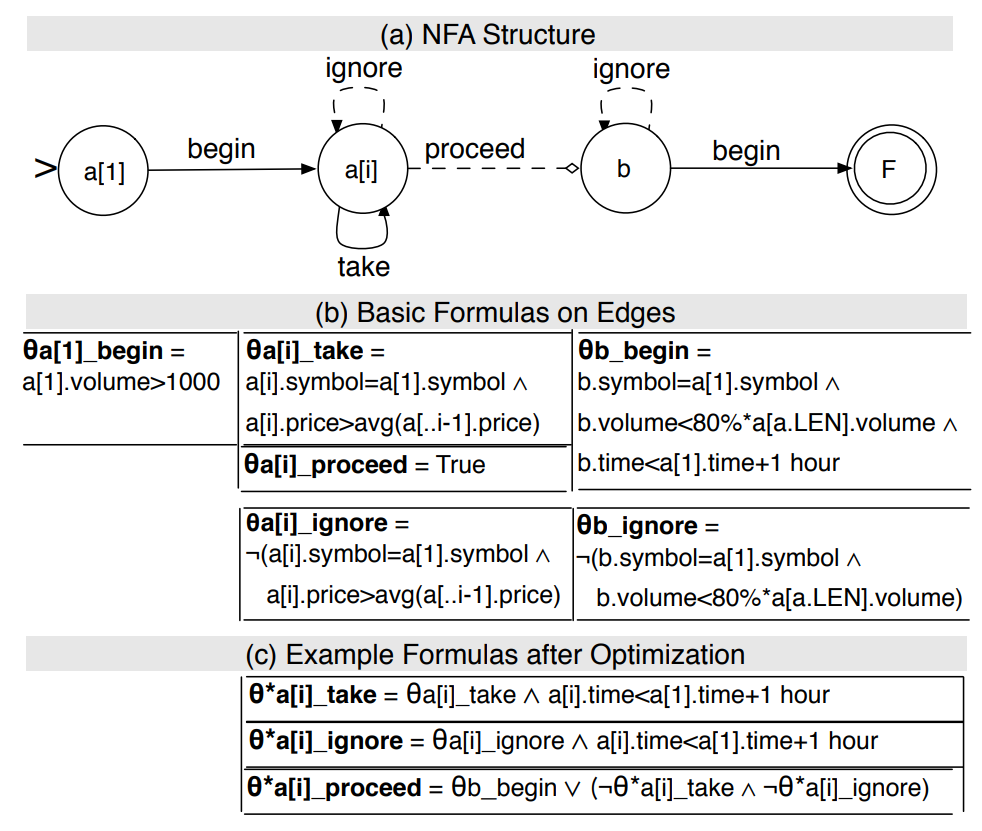$NFA^b$模型由一个非确定$NFA$状态机和一个匹配缓冲区组成。

$NFA^b$状态机的数学表达形式如下：

$A$ $=$ $(Q,E,\theta,q_1,F)$

$Q$ 状态的集合

$E$ 边的集合

$\theta$ 边上的条件计算公式

$q_1$起始状态

$F$结束状态

### 边

• 计算公式

计算公式用来计算匹配条件，记做 $\theta_{q_edge}$。计算公式是从模式查询语句编译而来。

• 对输入流的操作

是否消费输入的事件

• 对匹配缓冲区的操作

是否写入匹配缓冲区

• 实现表示begintake边，消费事件并写入匹配缓冲区。
• 虚线表示ignore边，消费输入事件但是不写入匹配缓冲区。
• 带菱形箭头的边是proceed边，不消费任何输入事件，根据边上计算公式判断是否推进到下一个状态。

### $NFA^b$运行实例

$NFA^b$实例到达结束状态的时候，就认为​匹配成功。模式查询就是在流上找到成功的模式匹配事件，并将结果输出。

## 查询编译$NFA^b$

### 基本算法

Query3为例逐步介绍该算法的基本步骤：

1. $NFA^b$结构

1. Predicates断言

1. 事件选择策略

ignore边上的的公式取决于使用的事件选择策略。尽管模式查询语义可能使用多个策略，但是我们的算法以简单、系统的方式确定状态q的ignore边$\theta_{q\_ignore}$的公式：

• 当使用严格相邻策略时，$\theta_{q\_ignore}$为False，不允许任何忽略任何事件。
• 当使用分区相邻策略时，$\theta_{q\_ignore}$的公式为¬ (partition condition)，允许忽略与该分区不相关的事件。
• 当使用跳过直到下一个匹配策略时，$\theta_{q\_ignore}$边的公式为¬ (take or begin condition)。回顾Query3，在图2（b）中，状态a[i]的ignore$\theta_{a[i]\_ignore}$的公式设置为$\neg\theta_{a[i]\_take}$，所有不满足take条件的事件都被忽略。
• 当使用跳过直到任意匹配策略时，$\theta_{a[i]\_ignore}$永远为True，允许忽略任何事件，包括相关和不相关的事件。
1. 事件窗口

最后，在指向结束状态的begin或者proceed边上，为整个状态赋予WITHIN中设定的事件条件。该断言判断第一个和最后一个匹配事件之间的时间长度是否超过了限定时长。

1. 时间窗口前推

到目前为止WITHIN条件放置在指向结束状态$F$的边上，可以将该条件放置到所有前序状态的takeignorebengin边上。这样可以在早期就能发现匹配时长超过了窗口限制，尽早的终止匹配，避免无效的计算。

在所有的边上都增加了时间判断条件，可能会增加一点计算资源的消耗，相对于无效计算而言，这点消耗是值得的。

图2（c）中表明了Query3中经过优化的$\theta_{a[i]\_take}$$\theta_{a[i]\_ignore}$

2. 约束proceed边

Query3中，proceed边的计算公式为True，后续的状态不是结束状态。状态a[i]的proceed边会导致不确定性，再创建一个新的$NFA^b$状态机实例

为了避免创建non-viable$NFA^b$实例，我们通过在当前事件中“窥视”并确定它是否能满足下一个状态b的begin边的条件来决定是进入下一状态。

在否定语义中，不允许proceed到下一个状态。有一个小的例外，如果a[i]的takeignore边的计算结果都是False，那么可以尝试proceed到状态b。

图2（c）是优化后的状态a[i]的takeignoreproceed边的条件。

在导致不确定性的边的位置，会导致创建新的$NFA^b$实例，用来表示不同的匹配。（此处需要仔细研究确认）

在本文中模式匹配语法使用的SASE+语法，但是算法的基本步骤1-4和优化步骤5-6同样适用于其他模式匹配语言。

## $NFA^b$的语义表达能力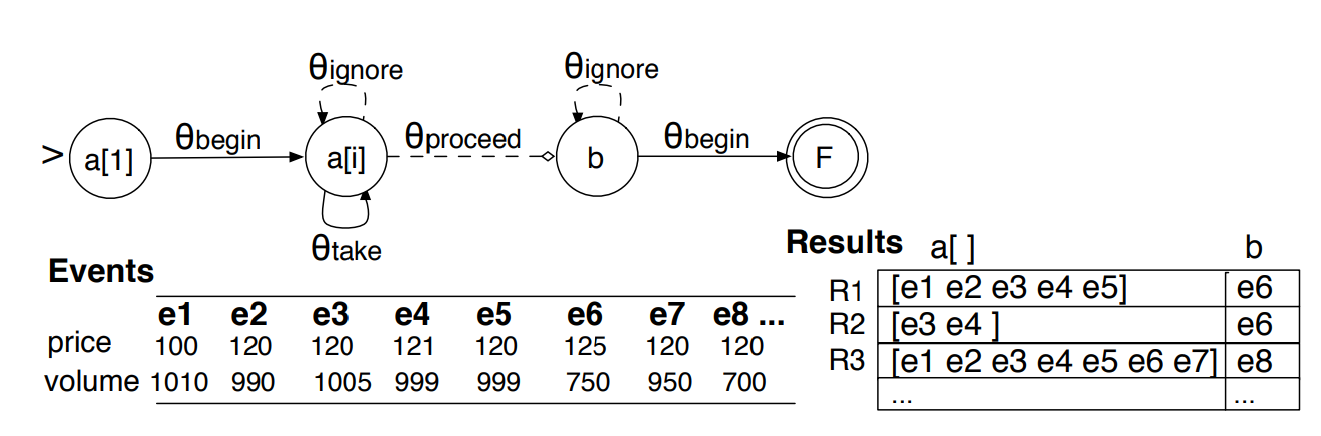# 运行时的复杂性

## 运行时的关键问题

### 并发的$NFA^b$实例

1. 满足开始条件创建实例

当新来的时间满足开始状态的条件时，创建一个新的$NFA^b$实例，新的实例与之前的$NFA^b$实例相互独立，没有关联关系。如图3中所示，事件$e_1$$e_3$都满足开始状态的条件，所以创建了两个$NFA^b$实例，分别生成了匹配结果$R_1$$R_2$

2. 非确定性边带来的新实例

• Take-Proceed

在图3中。当事件$e_6$到达状态a[i]时，$e_6$同时满足$\theta_{a[i] \_take}$$\theta_{a[i] \_proceed}$，此时有两个方向可以前进，此时会将状态机分裂成两个，分别指向不同的方向，这两个状态机，最终产生了不同的匹配结果，在图3中分别对应$R_1$$R_3$

Take-proceed这种不确定性，是由于查询匹配语句中的条件决定的，即便是采用严格相邻策略和分区相邻策略也不可避免。

• Ignore-Proceed

当使用比较宽松的策略skip-to-next-match时，$\theta_{a[i] \_ignore}$的条件也变宽松（如原文3.2所述）。在这种情况下，如果$\theta_{a[i] \_proceed}$$\theta_{a[i] \_ignore}$的条件不互斥，就会在ignore边和proceed边之间产生不确定性，图3中就有Ignore-Proceed不确定性。

• Take-Ignore

当使用了skip-till-any-match事件选择策略时，$\theta_{a[i] \_ignore}$永为True的时候。状态a[i]的take边和ignore边之间会产生不确定性。

• Begin-Ignore

当使用了skip-till-any-match事件选择策略时，任何孤子状态或者闭包状态的第一个状态的begin边和ignore边之间，都可能存在不确定性。

## 复杂性分析

### 复杂性证明过程

$T$ ：模式匹配查询语句中的时间窗口。

$C$：可以拥有相同时间戳的最大事件数量。

$p$：一个分区实例，$p$可以有1个或者多个（严格相邻策略把整个事件流视为1个分区）。

$W$：分区窗口的大小，可以使用$TCp$来估算。

### 共享的重要性

1. viablenon-viable $NFA^b$实例之间的共享

viable实例最终会到达结束状态，产生匹配结果。non-viable实例在匹配的过程中产生，但是最终会匹配失败。在 viablenon-viable 实例之间共享存储和计算资源，可以将所有的$NFA^b$实例的数量降低到匹配成功的$NFA^b$的数量。

non-viable $NFA^b$占大多数的时候，性能的提升尤其明显。

1. viable$NFA^b$实例之间的共享

### 匹配结果的输出成本

• bose 模式

枚举所有的事件，每个$NFA^b$实例输出各自的匹配的所有结果对象。匹配结果中包含了各自匹配到的事件对象。

• 压缩模式

返回匹配的引用的集合，集合中使用紧凑的数据结构（事件对象的引用，而不是存储事件对象本身）。提供解压缩算法，从共享缓冲区提取需要的事件对象。这样就可以避免一次性输出所有的匹配结果，而是让应用选择提取哪些对象，带来更大的灵活性。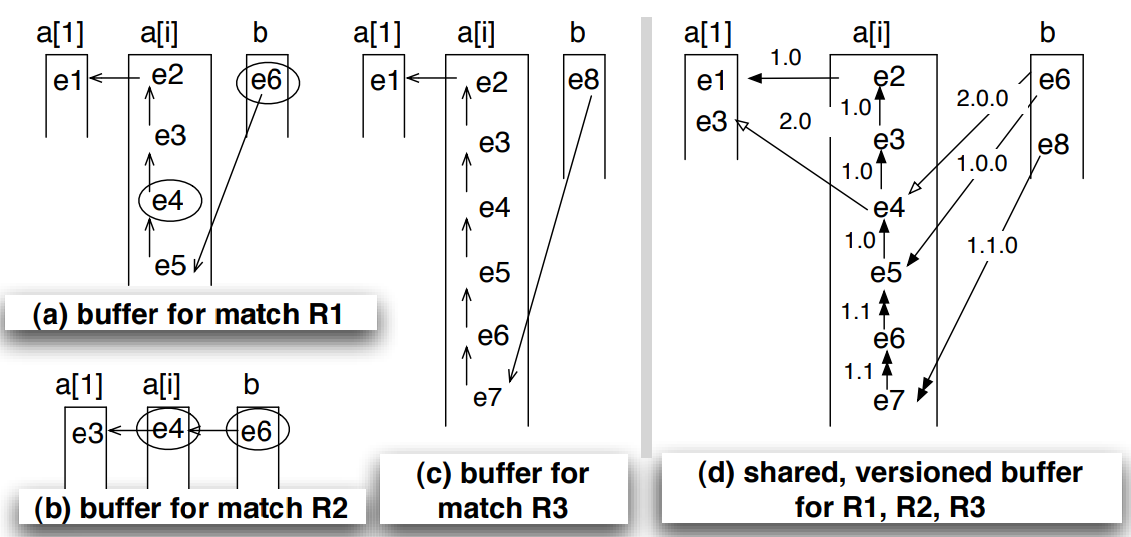​ 图4 Query3的匹配缓冲区示例

# 运行时的优化方法

## 基于版本的共享匹配缓冲区

$id_1$(.$id_j$)$∗$ $(1 ≤ j ≤ t)$

$t$指向当前的状态$q_t$

1. $v$使用$v^{'}$作为前缀
2. $v$$v^{'}$只有最后一位$id_t$不同，并且 $v$$id_t$ > $v^{'}$$id_t$

## 多个NFA实例的运行

• 模式匹配阶段

在模式匹配阶段，根据输入的事件流不断的判断是否要跳转到下一个状态，直到到达最终的结束状态。在匹配的过程中，把匹配的事件不断的写入到匹配缓冲区，以便在匹配结果构建阶段，生成完整的匹配结果。

• 匹配结果构建阶段

在匹配结果构建阶段，从匹配缓冲区取回该实例匹配的所有事件（上节中讲了）。

### 基本算法

computation state 是用来计算后续的边的公式（边上的where条件）的最小值的集合。

Query3为例，在状态a[i]上，计算take边的条件需要之前的价格的平均值$avg(a[..i − 1].price)$。缓冲区可以用来从缓存的事件中计算类似的值，但是有时候并不是最高效的做法。在本文中的做法是，牺牲一点空间效率换取性能，在匹配缓冲区之外，开辟一个很小的空间来保存计算过程中所需要的中间数据。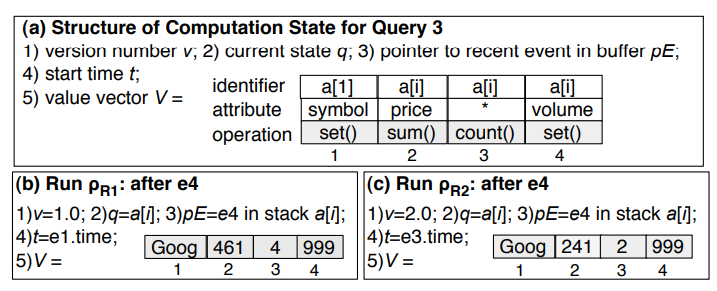​ 图5 Query3对应的 computation state

1. $NFA^b$实例的版本号 $v$
2. $NFA^b$实例所处的状态 $q$
3. $NFA^b$实例所对应的最新的事件的指针
4. $NFA^b$实例的起始时间
5. 一个向量 $V$，保存所有在未来的边的条件计算中需要用到的数据。

1. 向量$V$中的第2个列表示在状态a上，当有匹配的事件时，将事件的symbol属性记录下来，在后续的边等值条件判断中会用到。
2. 向量$V$中的第2、3列共同完成 $avg(a[..i − 1].price)$的计算，对于状态a，每一个匹配的事件，执行引擎获取事件的price的属性，维护一个求和后的总值sum，第3列维护事件的总的数量count
3. 向量$V$中的第4列保存状态a[i]的最后一个匹配事件的volume属性的值，用来计算跟状态b相关联的边的条件。

### 合并等价的$NFA^b$实例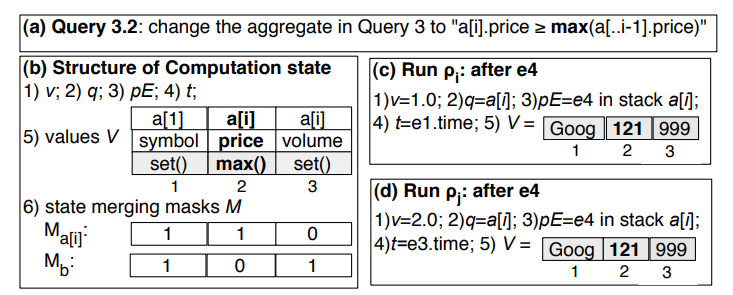​ 图6 $NFA^b$实例合并示例

1. 检测两个$NFA^b$实例是否等价

可能会在起始状态之后的任何一个状态$q_t$上进入等价。向量$V$完全一样的要求太严格，因为向量中的前边状态使用的中间数据，在后续计算中不再需要了。换句话说，只要在状态$q_t$和后续状态中需要的数据数据的值一样即可认为是向量$V$是等价的。

为了实现向量的等价判断，我们引入了一个新的静态字段$M$，如图6（b）所示，包含了一组bit标识位用来标记向量$V$。每一个标识位对应于一个状态$q_t$，该标识位用来表示该中间结果列是否与当前状态的计算相关。在实际运行的时候，可以根据该标识位获得($M_{qt}$ $\vee$ $M_{qt+1}$ $\vee$ $...$ )与当前状态$q_t$相关的所有中间结果值，记为$V_{[t...]}$，如果两个$NFA^b$实例的$V_{[t...]}$相同则可以进行实例的合并。

2. 创建合并的$NFA^b$实例

中间数据将包含所有版本号的数据、起始时间（此句话待研究）。合并的$NFA^b$实例的版本号将被缓存起来，留待后续匹配结果构建阶段使用。要做到这一点，必须要保证能够区分合并前实例在匹配缓冲区的指针引用，能够正确的取回匹配结果。同样还需要跟踪合并的实例的起始时间，以便于能够处理超时的实例（之前提到过，对于超过指定窗口长度的匹配需要中止匹配）。

合并实例的起始时间问题

合并的实例可能会有不同的起始时间，超时时间也不尽相同，执行过程中，可能存在一个实例超时，另外的实例还在执行的情况。为了能够使合并的实例能够尽快的处理完毕，合并实例的起始时间设定为被合并实例中最大的时间。

例如实例1起始时间为1，实例2起始时间为2，那么合并之后的实例的起始时间为2。

这样就可以保证当合并实例超时中止的时候，被合并实例也一定是超时的。

最后，当合并实例到达结束状态的时候，只有未超时的被合并实例会触发匹配结果构建。

### 回溯算法

1. 如果输出模式是输出所有的匹配结果，则向前追溯
2. 如果只有只输出非重叠的结果，则无需追溯。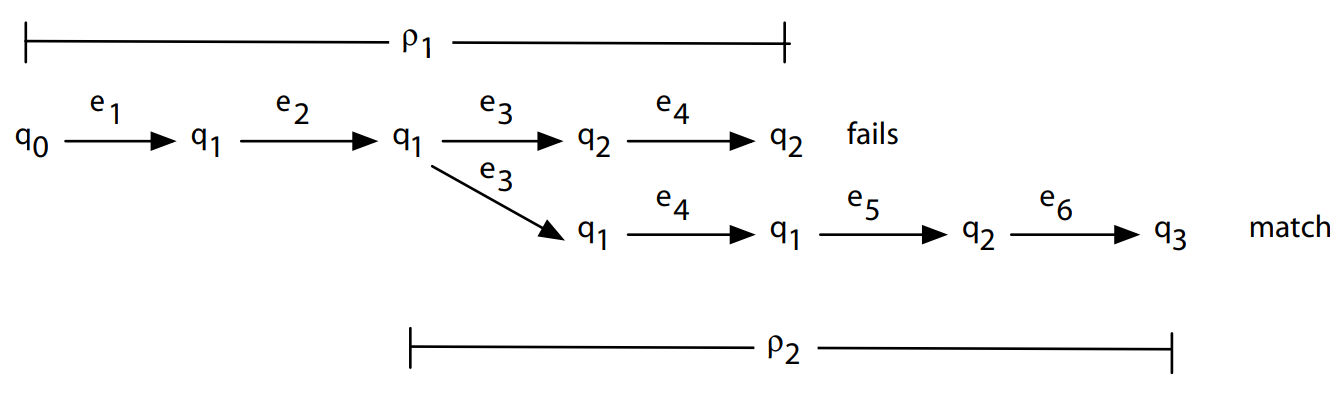​ 图7 回溯算法示例

1. $e_1$事件到来，在状态$q_0$创建一个$NFA^b$实例$\rho_1$$\rho_1$变成一个孤子实例。
2. $e_3$$q_3$上导致了不确定性，存在两条可能的前进的边。所以创建$NFA^b$实例$\rho_2$，将$\rho_2$、当前状态$q_1$的ID和当前事件$e_3$的ID一起添加到一个保存推迟运行的$NFA^b$实例的堆栈中。$\rho_1$继续作为孤子实例运行，因为$\rho_1$可以推进到下一个状态$q_2$，并没有失败或者匹配成功。
3. 继续处理$\rho_1$，直到当$e_4$到达时，在状态$q_2$匹配失败。
4. 向后回溯，从堆栈中弹出最新创建的$NFA^b$实例，在本例中是$\rho_2$。把$\rho_2$当做新的孤子实例，$\rho_2$从状态$q_1$开始执行，从事件$e_3$开始读取事件流。
5. $e_6$到达时，$\rho_2$匹配成功，输出匹配结果。

## 内存管理

• 匹配缓冲区

当事件被处理完毕之后，使用该事件的时间戳和时间窗口来确定位于窗口之外的最大时间戳，叫做清理时间戳。使用清理时间戳在匹配缓冲区的每个堆栈区域进行二分查找。通过二分查找确定确定最近的位于时间窗口之外的事件，从匹配缓冲区的堆栈中清理掉位于该时间戳和之前的所有事件。类似的，使用该时间戳，从全局的事件队列中清理掉事件。

• 重用频繁初始化的数据结构

当从匹配缓冲区中清除对象时，我们将它们添加到池中。 当请求新的堆栈对象时，我们首先尝试使用池中的任何可用对象，并仅在池为空时创建新的对象实例。 这样回收堆栈对象限制了对象实例化的数量并且减少了垃圾回收频率。

类似地，为$NFA^b$实例维护一个池，即保存中间状态数据的 computation state。 每当$NFA^b$匹配完成或失败时，我们将其添加到池中以便于重用。

# 性能评估